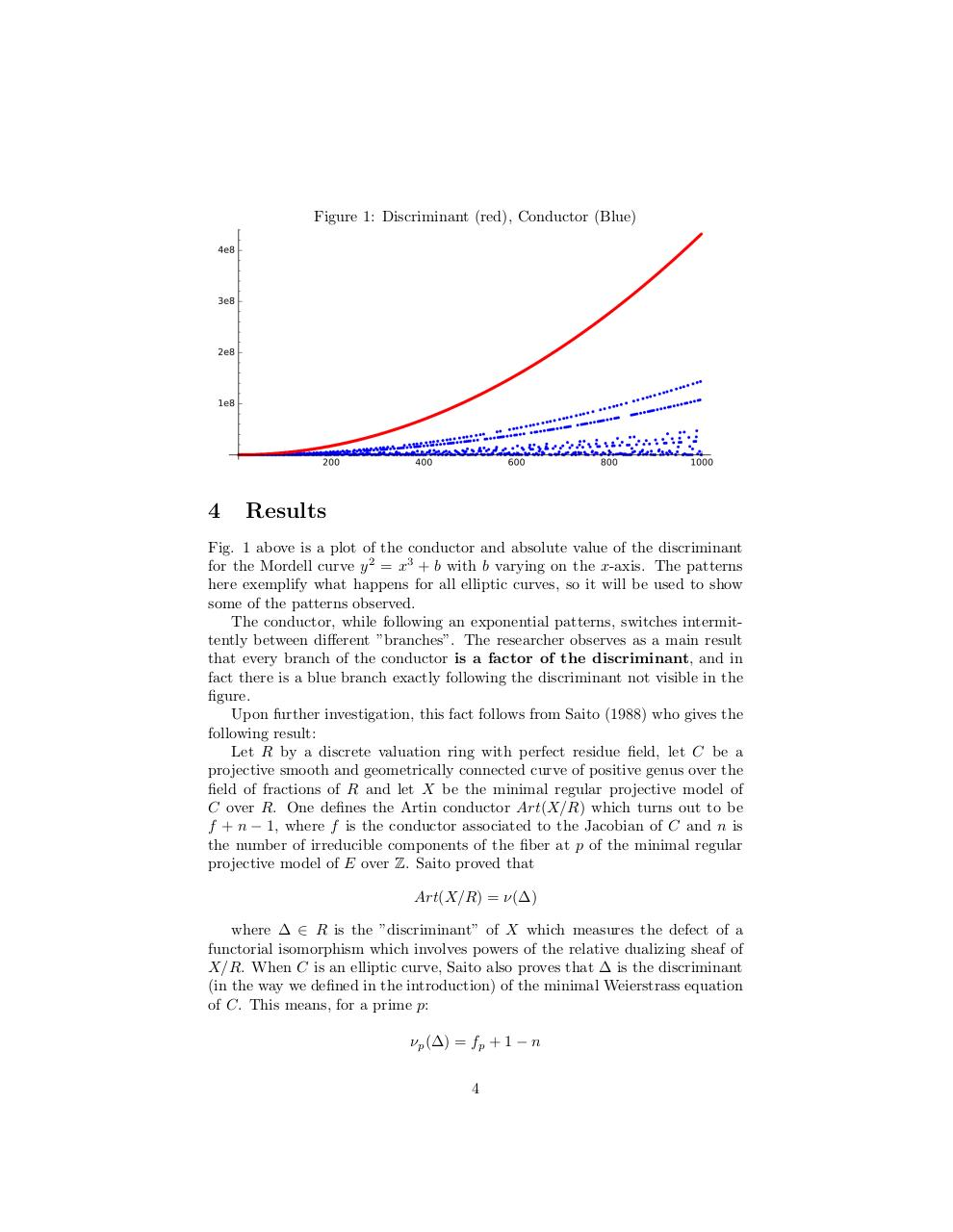# Sci Research (2).pdfPage 1 2 3 4 5 6 7 8 9

#### Text preview

Figure 1: Discriminant (red), Conductor (Blue)

4

Results

Fig. 1 above is a plot of the conductor and absolute value of the discriminant
for the Mordell curve y 2 = x3 + b with b varying on the x-axis. The patterns
here exemplify what happens for all elliptic curves, so it will be used to show
some of the patterns observed.
The conductor, while following an exponential patterns, switches intermittently between different ”branches”. The researcher observes as a main result
that every branch of the conductor is a factor of the discriminant, and in
fact there is a blue branch exactly following the discriminant not visible in the
figure.
Upon further investigation, this fact follows from Saito (1988) who gives the
following result:
Let R by a discrete valuation ring with perfect residue field, let C be a
projective smooth and geometrically connected curve of positive genus over the
field of fractions of R and let X be the minimal regular projective model of
C over R. One defines the Artin conductor Art(X/R) which turns out to be
f + n − 1, where f is the conductor associated to the Jacobian of C and n is
the number of irreducible components of the fiber at p of the minimal regular
projective model of E over Z. Saito proved that
Art(X/R) = ν(∆)
where ∆ ∈ R is the ”discriminant” of X which measures the defect of a
functorial isomorphism which involves powers of the relative dualizing sheaf of
X/R. When C is an elliptic curve, Saito also proves that ∆ is the discriminant
(in the way we defined in the introduction) of the minimal Weierstrass equation
of C. This means, for a prime p:
νp (∆) = fp + 1 − n
4